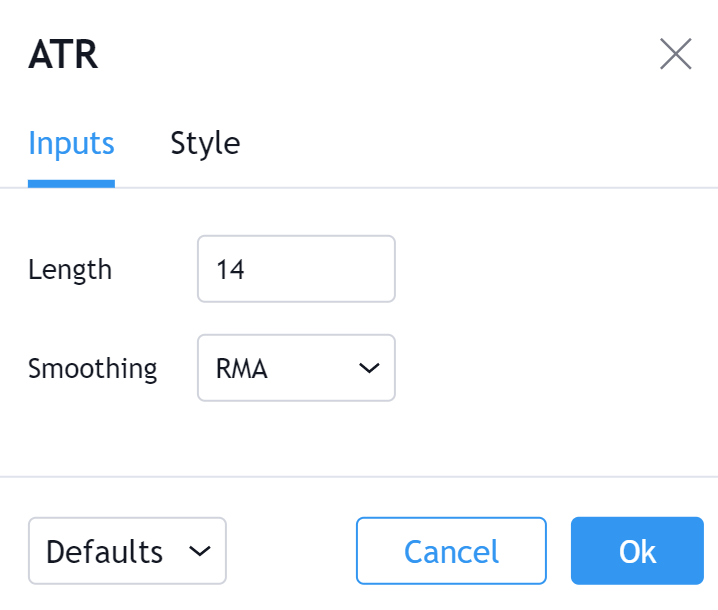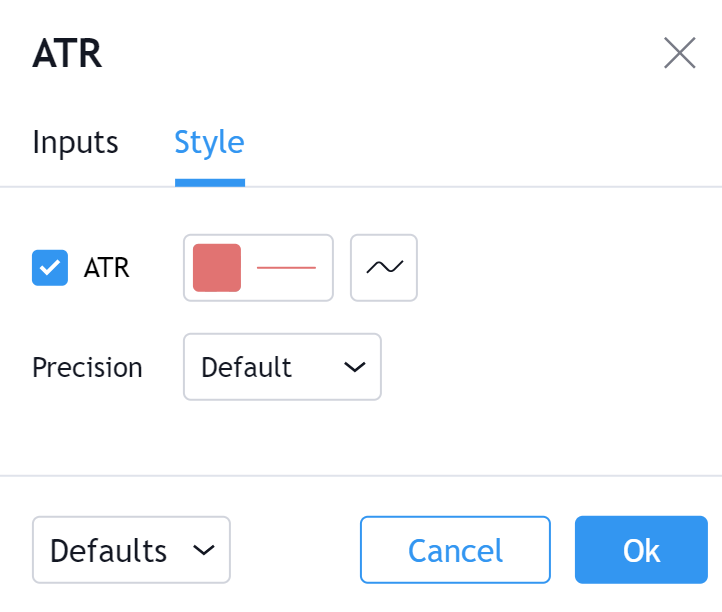# Average True Range (ATR)

#### Definition

The Average True Range (ATR) is a tool used in technical analysis to measure volatility. Unlike many of today's popular indicators, the ATR is not used to indicate the direction of price. Rather, it is a metric used solely to measure volatility, especially volatility caused by price gaps or limit moves.

#### History

J. Welles Wilder created the ATR and featured it in his book New Concepts in Technical Trading Systems. The book was published in 1978 and also featured several of his now classic indicators such as; The Relative Strength Index, Average Directional Index and the Parabolic SAR. Much like the indicators mentioned, the ATR is still widely used and has great importance in the world of technical analysis.

#### Calculation

To calculate the ATR, the True Range first needs to be discovered. True Range takes into account the most current period high/low range as well as the previous period close if necessary. There are three calculation which need to be completed and then compared against each other. The True Range is the largest of the following:

```The Current Period High minus (-) Current Period Low
The Absolute Value (abs) of the Current Period High minus (-) The Previous Period Close
The Absolute Value (abs) of the Current Period Low minus (-) The Previous Period Close```
`true range = max[(high - low), abs(high - previous close), abs (low - previous close)]`

*Absolute Value is used because the ATR does not measure price direction, only volatility. Therefore there should be no negative numbers. *Once you have the True Range, the Average True Range can be plotted. The ATR is an Exponential Moving Average of the True Range.

#### The basics

Average True Range is a continuously plotted line usually kept below the main price chart window. The way to interpret the Average True Range is that the higher the ATR value, then the higher the level of volatility.

• The look back period to use for the ATR is at the trader's discretion however 14 days is the most common.
• ATR can be used with varying periods (daily, weekly, intraday etc.) however daily is typically the period used.

#### What to look for

##### Measuring the Strength of a Move

As previously stated Average True Range does not take into account price direction, therefore it is not used as an active indicator to predict future moves. Instead, it is most useful in measuring the strength of a move. For example, if a security's price makes a move or reversal, either Bullish or Bearish, there will usually be an increase in volatility. In that case, the ATR will be on the rise. This can be used as a way to gauge the underlying strength of the move. The more volatility in a large move, the more interest or pressure there is reinforcing that move.

On the other hand, during periods of sustained sideways movement, volatility is frequently low. This could assist in the discovery of trading ranges.

##### Using Absolute Value

The fact that ATR is calculated using absolute values of differences in price is something that should not be ignored. This is relevant because it means that securities with higher price values will inherently have higher ATR values. Likewise, securities with lower price values will have lower ATR values. The consequence is that a trader cannot compare the ATR Values of multiple securities. What is considered to be a high ATR Value or a high ATR Range for one security may not be the same for another security. A trader should study and research the relevance of ATR for each security independently when performing chart analysis.

##### Compare the charts below.

Apple (AAPL) has a price over \$450 and an ATR over 12.

Ford (F) has a price over \$17 and an ATR of less than 1.

#### Summary

ATR is a nice chart analysis tool for keeping an eye on volatility which is a variable that is always important in charting or investing. It is a good option when trying to gauge the overall strength of a move or for discovering a trading range. That being said, it is an indicator which is best used as a compliment to more price direction driven indicators. Once a move has begun, the ATR can add a level of confidence (or lack there of) in that move which can be rather beneficial.

#### Inputs##### Length

The time period to be used in calculating the Average True Range. 14 days is the default.

#### Style##### ATR

Can toggle the visibility of the ATR Line as well as the visibility of a price line showing the actual current value of the ATR Line. Can also select the ATR Line's color, line thickness and visual type (Line is the default).

##### Precision

Sets the number of decimal places to be left on the indicator's value before rounding up. The higher this number, the more decimal points will be on the indicator's value.